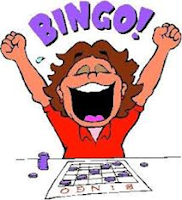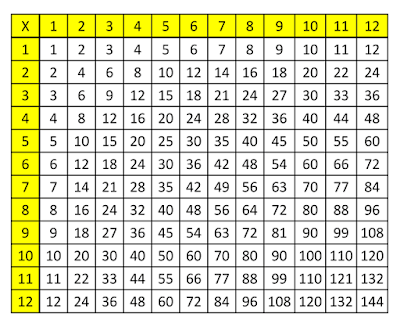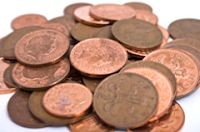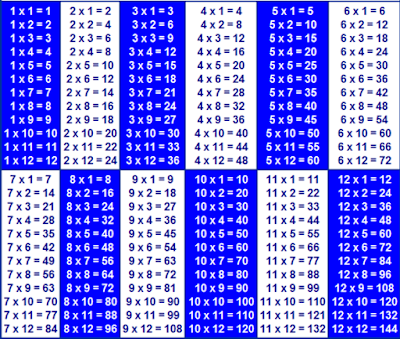Curriculum‎ > ‎Mathematics‎ > ‎Maths resources‎ > ‎

### Times Tables - games & resources

One of the most important things parents can help their children with is learning their multiplication tables. Spending time learning times tables is a brilliant way to help your child and it really helps speed up their mental maths.

Do you remember standing up, chanting tables at school? Learning by rote is one strategy, but there are also other
activities to help learn times tables. This booklet introduces some strategies that you could try at home to help your children learn their times tables.
Fast Fingers

This is a game for two players. The game is like rock, paper, scissors but with numbers. Two players count to 3 and then make a number using their fingers (one hand or two hands). Both players then have to multiply both numbers together. The quickest person wins!

 3 x 5 = 15

Multiplication BINGOThis game will need 2 players and a caller. Make a grid of six squares on a piece of paper and ask your child to write a number in each square from their target table. Give them a question and if they have the answer, they mark it off. First one to mark off all their numbers is the winner.
This game is even better if you have a bingo dauber.

Tricky Sixes

The six times table can be tricky to learn. One helpful trick is that in the 6 times tables, when you multiply an even number by 6, they both end in the same digit.

Double Up

The four times table. Double the number and double again, e.g.

3 x 4     double 3 = 6 double 6 = 12

6 x 4     double 6 = 12 double 12 = 24

Fingers of 9

2) For 9 x 4 bend your 4th finger down (like the picture).

3) You have 3 fingers in front of the bent finger and 6 after the bent finger. Thus the answer must be 36.

4) The technique works for the 9 times tables up to 10.

Multiplication SquareTimes Table SNAP

You will need a deck of cards for this game.
Flip over the cards as though you are playing snap. The first person to say the fact based on the cards turned over e.g. a 2 and a 3
(2 x 3 = 6) gets the cards.
The person who is left holding all of the cards wins.

Money Multiplication (x2, x5, x10)You will need a collection of 2p, 5p and 10p coins. Take a few coins and ask your child to use their times tables to work out the total value.

2 dice

Use stickers to change the numbers).
Roll the two dice and multiply the numbers.
If just practising certain multiplication tables, u
se the stickers to repeat the numbers on one of the die, e.g. 3 sides with 10 on and 3 sides with 2 on.

Once children know the times table facts in order, they can use flash cards to practise the facts out of order. They could just use them to answer questions, or for an extra challenge, try it against the clock. Flash cards could also be stuck around the house to help children learn the facts.

Sing a Song

Singing tables can be a really good way for children to learn. You can buy CDs of times table songs or download them from the internet that the children can sing along to, or you could always make up your own to a known tune.

Time to RhymeSilly rhymes can help to learn and remember tricky tables facts e.g.

 8x8 = 64 “He ate and ate and was sick on the floor, eight    times eight is 64" 3x3 = 9 “Swing from tree to tree on a vine, three times three is nine” 7x7 = 49 “Seven times seven is like a rhyme, it all adds up to 49.”

Patterns in numbers
Being able to spot the patterns in numbers is an important
skill and can also help with learning times tables. Children can investigate these multiplication rules:

Odd number x odd number = odd number (e.g. 3x5=15)

Even number x even number = even number (e.g. 4x6=24)

Odd number x even number = even number (e.g. 3x6=18)

Times Tables Lists